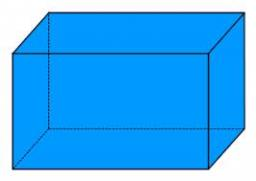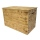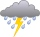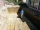# Cuboid

Cuboid with edge a=6 cm and body diagonal u=31 cm has volume V=900 cm3. Calculate the length of the other edges.

Correct result:

b =  30 cm
c =  5 cm

#### Solution:

$a=6 \ \text{cm} \ \\ V=900 \ \text{cm}^3 \ \\ u=31 \ \text{cm} \ \\ \ \\ V=abc \ \\ 900=6 \ bc \ \\ bc=150 \ \\ \ \\ u=\sqrt{ a^2+b^2+c^2 } \ \\ \ \\ 31^2=6^2 + b^2 + c^2 \ \\ \ \\ 925=b^2 + c^2 \ \\ 925=\dfrac{ 22500 }{ c^2 } + c^2 \ \\ c^4-925 \ c^2 + 22500=0 \ \\ x=c^2 \ \\ \ \\ x^2-925x + 22500=0 \ \\ \ \\ x^2 -925x +22500=0 \ \\ \ \\ a=1; b=-925; c=22500 \ \\ D=b^2 - 4ac=925^2 - 4\cdot 1 \cdot 22500=765625 \ \\ D>0 \ \\ \ \\ x_{1,2}=\dfrac{ -b \pm \sqrt{ D } }{ 2a }=\dfrac{ 925 \pm \sqrt{ 765625 } }{ 2 } \ \\ x_{1,2}=\dfrac{ 925 \pm 875 }{ 2 } \ \\ x_{1,2}=462.5 \pm 437.5 \ \\ x_{1}=900 \ \\ x_{2}=25 \ \\ \ \\ \text{ Factored form of the equation: } \ \\ (x -900) (x -25)=0 \ \\ \ \\ c_{1}=\sqrt{ x_{1} }=\sqrt{ 900 }=30 \ \\ c_{2}=\sqrt{ x_{2} }=\sqrt{ 25 }=5 \ \\ \ \\ b=c_{1}=30 \ \text{cm}$

Checkout calculation with our calculator of quadratic equations.Our examples were largely sent or created by pupils and students themselves. Therefore, we would be pleased if you could send us any errors you found, spelling mistakes, or rephasing the example. Thank you!

Please write to us with your comment on the math problem or ask something. Thank you for helping each other - students, teachers, parents, and problem authors.Tips to related online calculators
Looking for help with calculating roots of a quadratic equation?
Do you have a system of equations and looking for calculator system of linear equations?
Do you want to convert length units?
Tip: Our volume units converter will help you with the conversion of volume units.
Pythagorean theorem is the base for the right triangle calculator.

#### You need to know the following knowledge to solve this word math problem:

We encourage you to watch this tutorial video on this math problem:

## Next similar math problems:

• Identical cubesFrom the smallest number of identical cubes whose edge length is expressed by a natural number, can we build a block with dimensions 12dm x 16dm x 20dm?
• Cuboid to cubeA cuboid with dimensions of 9 cm, 6 cm, and 4 cm has the same volume as a cube. Calculate the surface of this cube.
• Wooden boxThe block-shaped box was placed on the ground, leaving a rectangular print with dimensions of 3 m and 2 m. When flipped over to another wall, a print with dimensions of 0.5 m and 3 m remained in the sand. What is the volume of the wooden box?
• Volume and surface areaFind the volume and surface of a wooden block with dimensions: a = 8 cm, b = 10 cm, c = 16 cm.
• Two rectangular boxesTwo rectangular boxes with dimensions of 5 cm, 8 cm, 10 cm, and 5 cm, 12 cm, 1 dm are to be replaced by a single cube box of the same cubic volume. Calculate its surface.
• Cuboid edgesCalculate the volume and surface of a cuboid whose edge lengths are in the ratio 2: 3: 4 and the longest edge measures 10cm.
• Cuboid and ratioFind the dimensions of a cuboid having a volume of 810 cm3 if the lengths of its edges coming from the same vertex are in ratio 2: 3: 5
• Block or cuboidThe wall diagonals of the block have sizes of √29cm, √34cm, √13cm. Calculate the surface and volume of the block.Calculate the volume (V) and the surface (S) of a regular quadrilateral prism whose height is 28.6 cm and the deviation of the body diagonal from the base plane is 50°.
• Aquarium heightHow high does the water in the aquarium reach, if there are 36 liters of water in it? The length of the aquarium is 60 cm and the width is 4 dm.
• Surface and volumeFind the surface and volume of a cuboid whose dimensions are 1 m, 50 cm, and 6 dm.
• Water tank300hl of water was filled into the tank 12 m long and 6 m wide. How high does it reach?
• RainGarden shape of a rectangle measuring 15 m and 20 m rained water up to 3 mm. How many liters of water rained on the garden?
• The excavationThe excavation for the city sewer was 38 m long, 2.2 m wide, and 3 m deep. a) How many cubic meters of soil were dredged b) How many journeys had to be made by one car when removing the soil, if they loaded an average of 4.5 m of cubic soil on each journe
• AquariumTry to estimate the weight of the water in an aquarium 50cm long 30cm wide, when poured to a height of 25cm. Calculates the weight of the aquarium's water.
• PoolHow many hl of water is in a cuboid pool (a = 25m, b = 8m) if the area of the wetted walls is 279.2 m2?
• Uboid volumeCalculate the cuboid volume if the walls are 30cm², 35cm², 42cm²